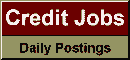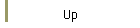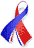DefaultRisk.com the web's biggest credit risk modeling resource.doi> search: A or B Export citation to:- HTML - Text (plain)- BibTeX - RIS - ReDIF

A Simplified Method for Calculating the Credit Risk of Lending Portfolios

by Akira Ieda of the Institute for Monetary and Economic Studies, Bank of Japan,
Kohei Marumo of the Institute for Monetary and Economic Studies, Bank of Japan, and
Toshinao Yoshiba of the Institute for Monetary and Economic Studies, Bank of Japan

December 2000

Abstract: The common practice for managing the credit risk of lending portfolios is to the calculate the maximum loss within the "value at risk" frame-work. Most financial institutions use large-scale Monte Carlo simulations to do this. However, such simulations may impose heavy calculation loads. This paper proposes a simplified method that approximates maximum loss with minimal simulation burden.

Our method divides a portfolio into subportfolios at each credit rating level and calculates the maximum loss of each subportfolio. We assume that the subportfolio's structure provokes little fluctuation in the ratio between the maximum loss and the standard deviation. We therefore begin with a subportfolio in which each exposure is of the same amount (a homogeneous subportfolio). Simple calculations provide the standard deviation for both the heterogeneous subportfolio whose risk is to be measured and the homogeneous subportfolio. The maximum loss for the homogeneous subportfolio can be obtained by using analytical techniques rather than simulations. The maximum loss for a heterogeneous subportfolio is then approximated by multiplying the ratio of the maximum loss and standard deviation of the homogeneous subportfolio by the standard deviation of the heterogeneous subportfolio. Simulation examples indicate that this approximation is effective in all portfolios except those including extremely large exposures. This paper also describes a technique for using the total maximum loss of all subportfolios to find the maximum loss for the entire portfolio.

Keywords: Credit risk, Lending portfolio, Monte Carlo simulation, Credit concentration/diversification, Correlation between default events.

Published in: Monetary and Economic Studies, Vol. 18, No. 2, (December 2000), pp. 49-82.